# How to use SUMIF function in Excel with formula examples

This tutorial explains the Excel SUMIF function in plain English. The main focus is on real-life formula examples with all kinds of criteria including text, numbers, dates, wildcards, blanks and non-blanks.

Microsoft Excel has a handful of functions to summarize large data sets for reports and analyses. One of the most useful functions that can help you make sense of an incomprehensible set of diverse data is SUMIF. Instead of adding up all numbers in a range, it lets you sum only those values that meet your criteria.

So, whenever your task requires conditional sum in Excel, the SUMIF function is what you need. A good thing is that the function is available in all versions, from Excel 2000 through Excel 365. Another great thing is that once you've learned SUMIF, it will take you very little effort to master other "IF" functions such as SUMIFS, COUNTIF, COUNTIFS, AVERAGEIF, etc.

## SUMIF in Excel - syntax and basic uses

The SUMIF function, also known as Excel conditional sum, is used to add up cell values based on a certain condition.

The function is available in Excel 365, Excel 2021, Excel 2019, Excel 2016, Excel 2013, Excel 2010, Excel 2007, and lower.

The syntax is as follows:

SUMIF(range, criteria, [sum_range])

As you see, the SUMIF function has 3 arguments - first 2 are required and the last one is optional.

• Range (required) - the range of cells to be evaluated by criteria.
• Criteria (required) - the condition that must be met. It may be supplied in the form of a number, text, date, logical expression, a cell reference, or another Excel function. For example, you can enter the criteria such as "5", "cherries", "10/25/2014", "<5", etc.
• Sum_range (optional) - the range to sum if the condition is met. If omitted, then range is summed.

Note. Please pay attention that any text criteria or criteria containing logical operators must be enclosed in double quotation marks, e.g. "apples", ">10". Cell references should be used without the quotation marks, otherwise they would be treated as text strings.

## Basic SUMIF formula

To better understand the SUMIF syntax, consider the following example. Suppose you have a list of products in column A, regions in column B, and sales amounts in column C. Your goal is to get a total of sales for a specific region, say North. To have it done, let's build an Excel SUMIF formula in its simplest form.

• Range - a list of regions (B2:B10).
• Criteria - "North" or a cell containing the region of interest (F1).
• Sum_range - the sales amounts to be added up (C2:C10).

Putting the arguments together, we get the following formula:

`=SUMIF(B2:B10, "north", C2:C10)`

or

`=SUMIF(B2:B10, F1, C2:C10)`

Both formulas only sum sales in the North region: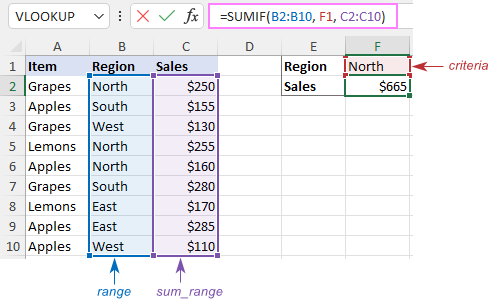Note. The sum_range parameter actually defines only the upper leftmost cell of the range to be summed. The remaining area is defined by the dimensions of the range argument. In practice, this means that sum_range argument does not necessarily have to be of the same size as range argument, i. e. it may have a different number of rows and columns. However, the top left cell must always be the right one. For example, in the above formula, you can supply C2, or C2:C4, or even C2:C100 as the sum_range argument, and the result will still be correct. However, the best practice is to provide equally sized range and sum_range.

Note. The SUMIF function is case-insensitive by nature. However, it is possible to force it to recognize the text case. For full details, please see Case-sensitive SUMIF in Excel.

## How to use SUMIF in Excel - formula examples

Hopefully, the above example has helped you gain some basic understanding of how the function works. Below you will find a few more formulas that demonstrate how to use SUMIF in Excel with various criteria.

### SUMIF greater than or less than

To sum numbers greater than or less than a particular value, configure the SUMIF criteria with one of the following logical operators:

• Greater than (>)
• Greater than or equal to (>=)
• Less than (<)
• Less than or equal to (<=)

In the table below, supposing you wish to add up the sales numbers for the items that ship in 3 or more days. To express this condition, put a comparison operator (>) before the number and surround the construction in double quotes:

`=SUMIF(C2:C10, ">3", B2:B10)`

If the target number is in another cell, say F1, concatenate the logical operator and cell reference:

`=SUMIF(C2:C10, ">"&F1, B2:B10)`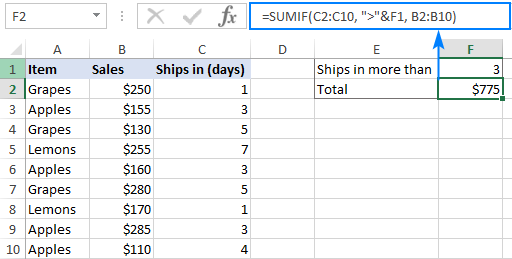In a similar manner, you can sum values smaller than a given number. For this, use the less than (<) operator:

`=SUMIF(C2:C10, "<3", B2:B10)`

### SUM IF equal to

A SUMIF formula with the "equal to" criteria works for both numbers and text. In such criteria, the equals sign is not actually required.

For instance, to find a total of the items that ship in 3 days, either of the below formulas will do:

`=SUMIF(C2:C10, 3, B2:B10)`

or

`=SUMIF(C2:C10, "=3", B2:B10)`

To sum if equal to cell, supply a cell reference for criteria:

`=SUMIF(C2:C10, F1, B2:B10)`

Where B2:B10 are the amounts, C2:C10 is the shipment duration, and F1 is the desired delivery time.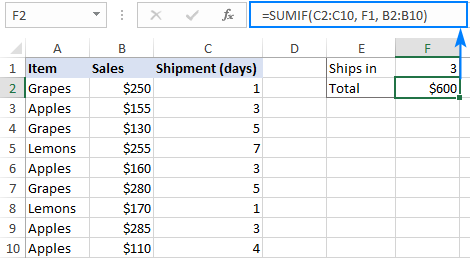Likewise, you can use the "equal to" criteria with text values. For instance, to add up the Apples amounts, choose any of the formulas below:

`=SUMIF(A2:A10, "apples", B2:B10)`

`=SUMIF(A2:A10, "=apples", B2:B10)`

`=SUMIF(A2:A10, F1, B2:B10)`

Where A2:A10 is the list of items to compare against the value in F1.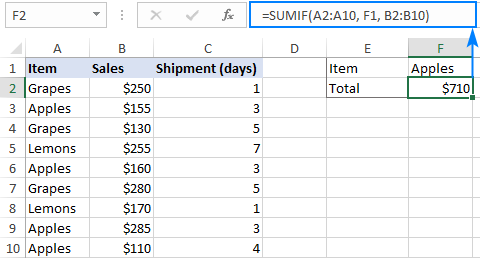The above formulas imply that the criterion matches the entire cell contents. Consequently, the SUMIF function will add up Apples sales but not, say, Green Apples. To sum partial matches, construct the "if cell contains" criteria like in this SUMIF wildcard formula.

Note. Please pay attention that, in Excel SUMIF formulas, a comparison or equals operator should always be enclosed in double quotes, whether used on its own or together with a number or text.

### SUM IF not equal to

To build the "not equal to" criteria, use the "<>" logical operator.

When a value, either text or number, is hardcoded in the criteria, remember to surround the entire construction with double quotes.

For example, to sum the amounts with shipment other than 3 days, the formula goes as follows:

`=SUMIF(C2:C10, "<>3", B2:B10)`

To find a total of all the items except Apples, the formula is:

`=SUMIF(A2:A10, "<>apples", B2:B10)`

When the criterion is in another cell, concatenate the "not equal to" operator and a cell reference like this:

`=SUMIF(A2:A10, "<>"&F1, B2:B10)`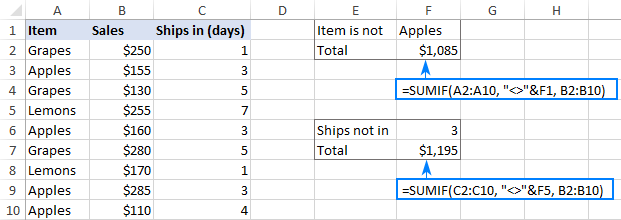### SUM IF blank

This example shows how to sum cells in one column if a corresponding cell in another column is blank. There are two formulas to fulfill the task. Which one to use depends on your interpretation of a "blank cell".

If "blank" means cells that contain absolutely nothing (no formula, no zero-length string returned by some other function), then use "=" for criteria. For example:

`=SUMIF(B2:B10, "=", C2:D10)`

If "blank" includes empty strings (for example, cells with a formula like =""), then use "" for criteria:

`=SUMIF(B2:B10, "", C2:D10)`

Both formulas return a total of sales for undefined regions, i.e. where a cell in column B is blank: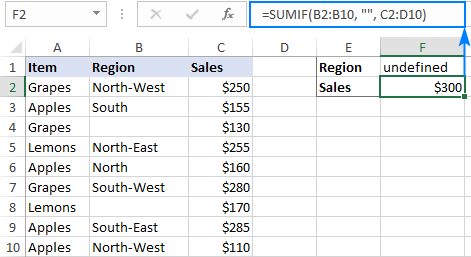### SUM IF not blank

To make "if cell is not blank then sum" kind of formula, use "<>" as the criteria. This will add up all cells that contain anything in them, including zero-length strings.

For instance, here's how you can sum sales for all the regions, i.e. where column B is not blank:

`=SUMIF(B2:B10, "<>", C2:D10)`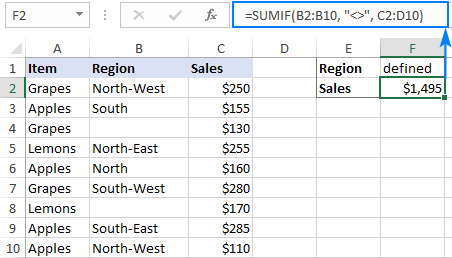### Excel SUMIF with text criteria

When adding up numbers in one column based on text values in another column, it's important to differentiate between exact and partial match.

Criteria Formula Example Description
Sum if equal to Exact match:
=SUMIF(A2:A8, "bananas", C2:C8)
Sum values in cells C2:C8 if a cell in column A in the same row contains exactly the word "bananas" and no other words or characters. Cells containing "green bananas", "bananas green", or "bananas!" are not included.
Sum if cell contains Partial match:
=SUMIF(A2:A8, "*bananas*", C2:C8)
Sum values in cells C2:C8 if a corresponding cell in column A contains the word "bananas", alone or in combination with any other words. Cells containing "green bananas", "bananas green", or "bananas!" are summed.
Sum if not equal to Exact match:
=SUMIF(A2:A8, "<>bananas", C2:C8)
Sum values in cells C2:C8 if a cell in column A contains any value other than "bananas". If a cell contains "bananas" together with some other words or characters like "yellow bananas" or "bananas yellow", such cells are summed.
Sum if cell does not contain Partial match:
=SUMIF(A2:A8, "<>*bananas*", C2:C8)
Sum values in cells C2:C8 if a cell in column A does not contain the word "bananas", alone or in combination with any other words. Cells containing "yellow bananas" or "bananas yellow" are not summed.

For real-life formula examples, please check out Sum if equal to and Sum if not equal to.

In the next section, we'll take a closer look at SUMIF formulas with partial match.

## SUMIF formulas with wildcard characters

To conditionally sum cells by partial match, include one of the following wildcard characters in your criteria:

• Question mark (?) to match any single character in a specific position.
• Asterisk (*) to match any number of characters.

### Example 1. Sum values based on partial match

Suppose you wish to total sales for all northern regions, including North, North-East, and North-West. To have it done, put an asterisk right after the word "north":

`=SUMIF(B2:B10, "north*", C2:D10)`

An asterisk on both sides will also work:

`=SUMIF(B2:B10, "*north*", C2:D10)`

Alternatively, you can type the region of interest in a predefined cell (F1), and then concatenate a cell reference and a wildcard character enclosed in quotes:

`=SUMIF(B2:B10, F1&"*", C2:D10)`

Or

`=SUMIF(B2:B10, "*"&F1&"*", C2:D10)`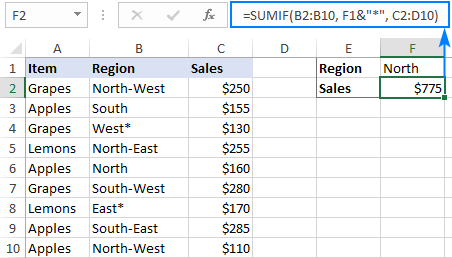### Example 2. Sum if cell contains * or ?

To match a literal question mark or asterisk, place a tilde (~) before the character, e.g. "~?" or "~*".

For example, to sum sales for the regions marked with *, use "*~*" for criteria. In this case, the first asterisk is a wildcard and the second one is a literal asterisk character:

`=SUMIF(B2:B10, "*~*", C2:D10)`

If the criteria (* in our case) is entered in a separate cell, then concatenate a tilde and the cell reference, like this:

`=SUMIF(B2:B10, "*"&"~"&F1, C2:D10)`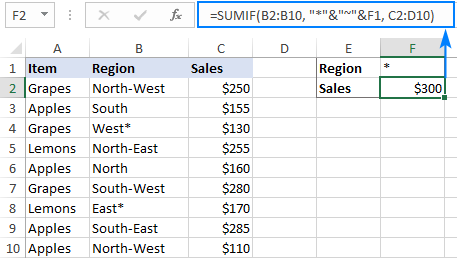### Example 3. Sum if another cell contains text

If your dataset contains various data types and you only want to sum cells corresponding to text values, the following SUMIF formulas will come in handy.

To add up values in cells C2:C8 if a cell in column A contains any text character(s):

`=SUMIF(A2:A8,"?*", C2:C8)`

To sum values in C2:C8 if a cell in column A contains any text value, including zero length strings:

`=SUMIF(A2:A8,"*", C2:C8)`

Both of the above formulas ignore non-text values such as numbers, dates, errors, and Booleans.

## How to use Excel SUMIF with dates

Using dates as SUMIF criteria is very much like using numbers. The most important thing is to supply a date in the format that Excel understands. If you are not sure which date format is supported and which is not, the DATE function can be a solution.

Assuming you are looking to sum sales for the items delivered before 10-Sep-2020, the criteria can be expressed in this way:

`=SUMIF(C2:C10, "<9/10/2020", B2:B10)`

or

`=SUMIF(C2:C10, "<"&DATE(2020,9,10), B2:B10)`

or

`=SUMIF(C2:C10, "<"&F1, B2:B10)`

Where F1 is the target date.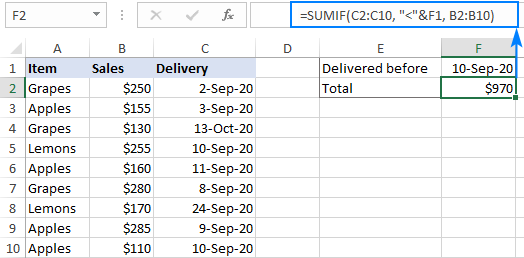To sum cells based on today's date, include the TODAY function in your criteria. For example, that's how you calculate a total of sales with a delivery date prior to today:

`=SUMIF(C2:C10, "<"&TODAY(), B2:B10)`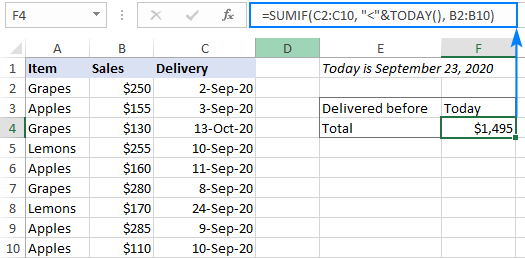To sum within a date range, you need to define a smaller and larger date separately. This can be done with the help of the SUMIFS function that supports multiple criteria.

For example, to sum values in column B if a date in column C is between two dates, this is the formula to use:

`=SUMIFS(B2:B10, C2:C10, ">="&F1, C2:C10, "<="&G1)`

Where B2:B10 is the sum range, C2:C10 is the list of dates to check, F1 is the start date and G1 is the end date.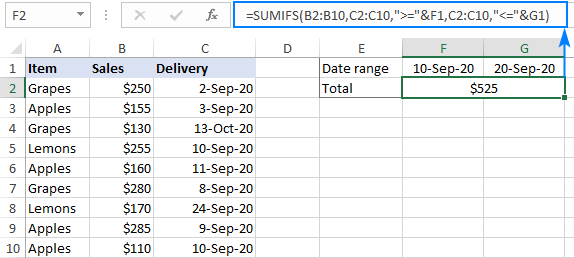More formula examples can be found in SUMIFS with date range as criteria.

## How to do SUMIF from another sheet

To conditionally sum data from a different sheet, provide external references for the SUMIF arguments. The easiest way is to start typing a formula, switch to another worksheet and select ranges using the mouse. Excel will insert all the references automatically, without you having to worry about the correct syntax.

For instance, the below formula will add up values in C2:C10 on the Data sheet based on the criteria in B3 on Sheet1:

`=SUMIF(Data!B2:B10, B3, Data!C2:C10)`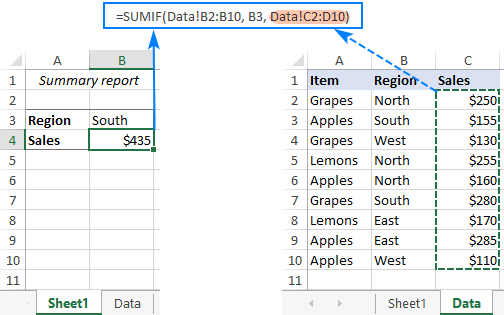To create a flexible formula, you normally insert all variable parameters in predefined cells instead of "hardcoding" them. With Excel SUMIF, that might be a bit of a challenge.

In the simplest case when summing "if equal to", you simply use a cell reference for criteria. For example:

`=SUMIF(C2:C10, F1, B2:B10)`

But when a cell reference is used together with a logical operator, the criteria should be provided in the form of a string. So, you use the double quotes ("") to start a text string and ampersand (&) to concatenate and finish the string off. For example:

`=SUMIF(C2:C10, ">"&F7, B2:B10)`

Please note that the comparison operators are enclosed in quotation marks while the cell references are not.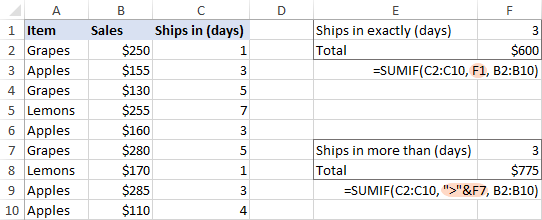1. I am trying to display a total (sum) from 3 cells which haven't had data entered yet so they are currently blank. I don't want the result to be a "0" unless information entered into any of these 3 cells total "0", I want the result to be blank if all 3 cells are blank.

• Hello!
To avoid showing 0 in a cell, you can use a custom number format. I recommend this guide: Display zeros as dashes or blanks.
You can also check for empty cells with the ISBLANK function and use this as a condition in the IF function.
The formula might look like this:

=IF(SUM(--ISBLANK(A1:A3))=3,"",SUM(A1:A3))

2. I have data in column B2:B100 describing a sinusoidally oscillating signal oscillating about 0. The amplitude of the oscillations are either increasing or decreasing amplitude, and I want to find:

1) the values of the Peaks in column F (like E1says "first Peak" and F1 contains magnitude of first peak.
2) the values of the Valleys in column H (like G1says "first Valley" and H1 contains magnitude of first Valley.

How do I set up the excel statements? Appreciate your help.

Yogi Dayal

3. Hi , I'm trying to make a formula for today profit , so it updates every day based on today date I'm putting it as =sumif(range,"today()",sum_range And for sum reason it always shows \$0 , do you know what the formula should be in this case?

• Hello!
Please check out this article to learn how to sum data based on today or between two dates.

4. I want Cell D17 to perform equation (D21-C21*100) but only if E21 and E22 are >=100%.

• Hi!
To use multiple conditions in a formula, use the AND operator in the IF function. In this article, you will find a detailed description and examples.

IF(AND(E21 >=100%, E22 >=100%), D21-C21*100,"")

5. Hi I am in the process of completing MOS 201 Objective 3.1. Not sure if you are familiar with the book but I cannot seem to figure out this sub objective. I tried different ways and I had gotten the answers but I am still getting (DiV/0) which should not be.

On the Gross Margin worksheet, add formulas to the Gross Margin field (H7:H14) that calculate the gross margin by subtracting Cost from Retail and then dividing by Cost. To avoid #DIV/0! (division by 0) errors, wrap the calculation inside an IF function that returns the gross margin if Cost is not 0, or the message Cost is 0! otherwise.

6. trying to create a Gannt chart. part of this is a list of tasks and sub tasks which are assigned numbers e.g 3 and this might have a sub task 3.1 which again may have a sub task 3.1.1
to each task or sub task is assigned a duration in days. the duration will be assigned to the lowest sub task in this case task 3.1.1 So i want a formula that will sum up the duration for the subtasks that fall under its "jurisdiction" so for subtask 3.1 it would include durations assigned to task 3.1.1, 3.1.2.... 3.1.n but not any time associated with any other subtasks under task heading like 3.2 or 3.2.1 or 4 or 4.1.
i would like the formula to be able to be dragged to autofill cells beneath and the formula still work

• Hello!
If your list of tasks is sorted, you can use the SUBTOTAL function. You can also use the recommendations in this article: SUMPRODUCT with multiple criteria. The formula might look something like this:

=SUMPRODUCT(--ISNUMBER(SEARCH(A2,A3:A10)),B3:B10)

7. I need to sum a vast list but only three columns. A is the technician name, B is the cost of labor, C is the invoice. One invoice can have a dozen entries and I just need the total for that invoice. I'm a novice, thanks.

JOE SMITH 25.00 338117
JOE SMITH 5.00 338117
JOE SMITH 12.50 338118
JOE SMITH 262.50 338150
JOE SMITH 57.50 338160
JOE SMITH 12.50 338160
JOE SMITH 7.50 338160
JOE SMITH 5.00 338160
JOE SMITH -2.50 338160
JOHN JONES 22.50 338161
JOHN JONES 12.50 338161
JOHN JONES 10.00 338161
JOHN JONES 5.00 338161

• Hello!
Use the SUMIF function to calculate the invoice amount:

=SUMIF(C3:C10,338117,B3:B10)

• Thank you, I would have to enter it for every invoice? I'm simply highlighting each section to get the total now.

• Hello!
If you just want the total amount and not the sum of each account, use the SUM function.

8. I want to sum the values in column B which satisfies that any data in column A is greater than 7 but less than 13.
How do i write the formular?
Kindly help.
Thanks.

• Hi!

9. i want to multiply 2700 if total days is less than 14 and greater than equal to 14 is multiply by 4000 please help

• Hi!
You haven't written that you want to multiply. But you need to use the IF function to do the multiplication depending on the conditions.

10. My issues is that I need to calculate several cells together but only if a name exists in the first cell of the row,
B C D E F G H
12 kl 50 28 13 18.1 50.5. TOTAL

so B12 would be a name and if no name appears in that cell then there is no total

• Hello!
To find the sum for a row with a condition, use something like the following formula

=IF(B12="Name",SUM(C12:H12),"")

If this is not what you wanted, please describe the problem in more detail.

• this is what I am working with

B19 c19 D19 E19 F19 G19 H19 I19 J19 K19 L19 M19 O19 P19 Q19
JOSH 50 28 13 18.1 50.5 10 20.50 9.16 =sum of c19:p19

meg 50 28 13 18.1 50.5 10 20.50 9.16 =sum of c20:p20

I need the sum of c:q for the total of monthly fees. I only want it to total if there is a name in the B column. The name will change in each row.

I tried the first formula and I am getting a blank space

• Hello!
Change the condition in the formula:

=IF(B12<>"",SUM(C12:P12),"")

11. =SUMIFS(G22:G114,\$K\$22:\$K\$114,2022)

What if I want to take the value in G:22:G114 and divide it by a number in another column, say N:22:N114, before adding.

The value in the N could be different for each row. So in this example if the value for G26 is 1000 and the value for N26 is 4 then it would only add 250

The next row could have a value of 400 in the G column and a 2 in the N column and it would add 200.

So at this point I would have a value of 450.

• Hello!
If I understand your task correctly, the following formula should work for you:

=SUMPRODUCT(G22:G114/\$K\$22:\$K\$114)

12. How to use SumIF formula to calculate commission
Problem: Minimum Guarantee is 1000 or 10% of sales whichever is higher. Please explain how to use the formula. Thanks

13. I want just to thank you for explaining the use of asterisk in condition of SUMIF to find certain word in a string (e.g. "*apple*"). A discovery for me :-)

14. for Example
item id item name Qnty Stock
47158 Whirlpool Double Door Refrigerator 340L IF INV CNV 355 3S Arctic Steel 1 Yes

15. if i got cell A1 to AA1,but i only require to sum every 1,3,5,7,9....column
How should i set the sumif formula?

• Hello!

=SUMPRODUCT(\$A\$1:\$AA\$1*ISODD(COLUMN(\$A\$1:\$AA\$1)))

Hope this is what you need.

16. Is it possible ta change the cell color of two cells when these two cells are greater than 70% when summed together?

17. SUMIF question. I have this formula which works fine. =SUMIF(E4:E319,">.2",C4:C319). However, I would like the ">.2" part of the formula to be in a cell where you can change the value from .2 to whatever you would like. Is that possible?

• Hello!
Change the formula, replace the condition with a cell reference

=SUMIF(E4:E319,D1,C4:C319)

In D1 -- ”>.2″

18. I want to use sumif function where input range to evaluate is output of Vlookup function, but it does not work as the output of sumif is always coming "0"

• Hello!
I’m sorry but your task is not entirely clear to me. For me to be able to help you better, please describe your task in more detail. Please specify what you were trying to find, what formula you used and what problem or error occurred. Give an example of the source data and the expected result.
It’ll help me understand it better and find a solution for you.

19. Hi,
I have data of purchases of several different type of items on different dates and different quantities throughout the year. I want to apply such formula that I get a report in which it gives total purchase quantity for particular period, say Aug 15 to Oct 10, for the item written in A1 cells.

20. Sir,
Thank you. Please give one day. Tomorrow I will confirm.

21. Sir, Thank you very much for your support. My query is below.
10001 10003 10005 10006 10007 Total
7712 398 250 125 4700 13185
5784 299 250 325 4667 11325
1) I want to sum if the Header (Criteria) 10001,10005
2) I want to sum if the header "define name" - Criteria used defined name

• Hello!
If I understand your task correctly, the following formula should work for you:

=SUM(((A1:E1=10001)+(A1:E1=10005))*A2:E10)

22. I write sumifs function as below with multiple criteria. But showing error.

=SUM(IF(K1:BE1{"10001","10003"},K134:BE134,0))
=SUMIFS(K134:BE134,K1:BE1,"10001,10003")
=SUMIFS(K134:BE134,K1:BE1, hcode)
hcode as range
hcode contains 10001,10003

• Hello!
I hope you have studied the recommendations in the above tutorial.
Please specify what you were trying to find, what formula you used and what problem or error occurred.The first formula violates the syntax rules of the IF function. The data ranges in the SUMIFS function must be the same size. You also violated the syntax rules. Include an example of the source data and the result you want to get. It’ll help me understand the problem you faced better and help you.

23. Hi, I'd really appreciate your help with something.
I'm trying to work out the number of days between 2 dates, but if there is no date in column B, I would like column C to read "Not Seen". Here's an example of a date in column B and then no date in column B.
Column A Column B Column C
1/6/2020 6/6/2020 5 (using formula =B2-A2)
1/6/2020 -45778 (this is what's happening now)
1/6/2020 Not Seen (this is what I would like to have)

• Hello Bec!

=IF(B2<>"",B2-A2,"Not Seen")

24. =SUMIF(\$A\$17:\$A\$28,A2&">0",\$D\$17:\$D\$28) is it Correct ">0"

Plus Valuve Minus Valuve
01/07/2020 - -
02/07/2020 - -
03/07/2020 - -
04/07/2020 - -
05/07/2020 - -
06/07/2020 - -
07/07/2020 - -
08/07/2020 - -

01/07/2020 \$1,000.00
01/07/2020 \$2,000.00
01/07/2020 \$5,000.00
02/07/2020 \$(500.00)
02/07/2020 \$(10,000.00)

25. so I have a simple thing that for some reason I cannot make happen. I need to sum a range of cells, but I need to only show the value of the cell that is 12 or less in the cell, but still allow the next cell to show what is over 12.
this is a payroll issue to separated out overtime...
so.. we have 4 areas that employees can work hours, I need to total those 4 cells and have only the first 12 hrs actually show in the reg hours worked and then anything over 12 will show in the overtime.
Seems simple -- but so not for me!

• Hello Nancy!
If I understand your task correctly, in cell F1 write down the following formula:

=IF(SUM(B1:E1) > 12,12,SUM(B1:E1))

And in cell G1 write down the following formula:

=IF(SUM(B1:E1) > 12,SUM(B1:E1)-12,0)

26. Here Khata Column followed by "do" I want to add Area Field

Khata Plot Area Rent Cess
1 361 0.18 5.00 3.00
do 367 0.155 5.00 2.50
do 360 0.165 5.00 2.50
2 413 0.068 0.80 0.50
3 348 1.128 13.80 10.50
5 206 0.63 6.30 3.00
6 253 0.065 6.45 3.00
do 254 0.085 6.45 3.00
do 257 0.08 6.45 3.00
do 255 0.025 6.45 3.00
do 256 0.008 6.45 3.00
do 250 0.138 6.45 3.00
do 251 0.066 6.45 3.00
do 252 0.03 6.45 3.00

27. I need to calculate the following table
f 2
m 1
m 5
f 2
f 4
f 1
m 0
m 1
f 1
in this two columns, column 1 = m & f, column 2 = figures, i need how many 0-5 f's in figures column and 0-5 m's. no need the total need only the number of, please help

28. Hi, I am currently using the "SUMIF in combination with the TODAY()". I have a problem with the weekends. If I use the "<"&Today(), unfortunately on the 06.01.2020 no value is provided. Is there a way how i can exclude the weekends in the formula and still obtain the values? I wish to avoid changing the formula manually. I rather have a formula which I can drag and obtain the correct values. Thanking you in advance for your help.
03.01.2020
06.01.2020
07.01.2020
08.01.2020
09.01.2020
10.01.2020

29. =IF(AND(TEXT('Outbound data'!K:K,"0")="TFS-01.MATE,KHM.ALI",TEXT('Outbound data'!L:L,"0")="110018144"),SUMIF('Outbound data'!L:R,\$E5,'Outbound data'!R:R),"0")
I want to compare two places if two places value match then respective line next row value sum should appear.

I want to filter some text but I can,t. Please help in this matter. The need is.... I have pending customers list. And the Heading is "Serial NO., Name, Mobile No., Due month& Year".

I want filter the Year before 2017 and don't Show the customer Details entire the serial number linked with the Year I filter. Please help me.

31. hi i dont know if this is the right place to ask but. i have some columns that has either 1 or 0 in them and i need to add up the 1 up to the first 0 and no further.problem is there may be hundreds of 0 in the same column. and the same for 1. so my question is how can i add up all the 1 up to the first 0 and nothing else. ive been doing it manually but it is annoying plus the chance for error is bigger.

32. Sir I need a help.
I want to addition 2 column with a condition ie
need calculation in U4,condition is that if column no "N4" is >= column No F4, need 0 if not, need original amount while adding N4+T4

33. Sir I need a help.
I want to addition 2 column with a condition ie
need calculation in U4,condition is that if column no "N4" is >= column No F4, need 0 if not, need original amount while adding N4+T4

• hi,i am struggling with calculating data.
how to calculate if range of specified text is met then sum range greater than 0 in one cell.
eg. IF(A2:A50,"abc") THEN SUMIF(F2:J15,"<0")

Thank you for looking in my post.

34. Thanks for posting this! Great information and clearly presented!

35. Hello there,

I need a formula to do the following...
if column D contains today's date, and column F contains "apple" then SUM column E.

36. Thankyou so much for being help us. but here i want to ask how we can copy text statement from one sheet to another sheet so that if we changed in master sheet the change could appear in other sheet automatically.

37. Thank you so much for this site. I was able to finally figure out the function that I needed and how to write the different parts.

38. Just what I needed. Thanks for the detailed explanation.

I have a tracking table for tracking Design request enquires.
i need to track on MONTHLY basis total number of Design Requests with Status (open / Close) and Overdue (Yes / No)

40. Hi, how to combine both formulas in one cell?
=SUM(H31,I31,J31)
=IF(H31<I31,I31)+If(H31<J31,J31)
I need to sum 3 coloums (H,I,J) but if I & J have values then H will need to subtract.
Thanks & regards,
Amy

41. I wish to be able to allow a cell ( D6 )\$ amount to be included in the column ( D1 to D20 )total if the next cell ( E6 )is marked with a check symbol ( wingdings 2 ). It said cell ( E6 ) is marked with an x ( wingdings 2 ), then the cell ( D6 ) with the \$ is not included in total. And so on down the page for my monthly bills accounting.

42. I'm trying to develop a mileage tracking sheet for both personal and business entries. Column A is the odometer when I arrive at a certain location. Column B will have a "Y" if the mileage is personal in that row. Column C is the total miles driven since the last stop if it is for business, no "Y" in column B for that row. Column D is the total miles driven since the last stop if it is for personal, "Y" in the B column for that row. Cell H2 contains a running total of business miles for the curent month. Cell H4 contains a running total of personal miles for the current month. I can't figure out how to subtract, for example, cell A5 from cell A6 using the SUMIF or SUMIFS function. I think I can write a formula for a running total for personal and business miles driven, but if you can suggest a formula I would appreciate it. Do I need to write a formula or include in the formula where to place the total miles driven for any certain row in either column C or D? Meaning for business miles driven on a single drive, no "Y" in column B for that row, and place that number in column C for that row and leave column D for that row blank. As you can tell, I'm struggling with these formulas. Thank you in advance for your help. DB

43. Substantially important point below, don't think I've seen this nuance pointed out anywhere else!

"The point is that Microsoft Excel does not rely on the user's ability to provide matching range and sum_range parameters, so to avoid possible inconsistency issues and prevent errors, it determines the sum range on its own in the following way. It takes the top left cell in the sum_range argument as the beginning cell (C2 in our Excel SUMIF example), and then includes as many columns and rows as contained in the range argument (in our case, it's 1 column x 7 rows, i.e. C2:C8)."

44. So I'm running into some issues with a compound SUMIF formula.

=SUMIF(AND(A8:A200,G8:K200,">"&Q1,G8:K200,""&0)

The main issue is that there are missing values for the lines that have a 0 in the "count" column as the information is being pulled from another table where "Count" is compiled using a COUNTIF formula. This being the case, these rows have the #N/A value plugged in if the count column shows a 0 which prevents the data from calculating. Because of this, I am hoping someone may be able to help me out and suggest a way for me to add up the dollar values for any dates that appear in a column that is between Days 1 and 2.

Essentially I need it to do the following:

1. Check columns G for any dates within a 1week timeframe
2. add up the values that have a quantity of 1 or more.

does anyone have any suggestions?

I want to use several Sumifs and all work except for the following.

In column B there will be a list of numbers and I want the calculation to exclude all numbers that end with the number 2, is this possible? or the reverse add up all the numbers that don't end with the number 2.

Phil

46. How can i use Sumif statement with the condition or criteria is a highlighted cell? looking to pull cost based on Green, Yellow, or Red Cells. any help would be great.

47. hi,

i am maintaining invertory in excel, by the current date
opening stock has to come with the total of previous for specific item.
please guide the formula. my formate is as follows

item Description 19-Jun-18 20-Jun-18 21-Jun-18 22-Jun-18
Item No.1
Item No.2

48. I Want Help On following
Tax Slab Rate of Tax
- 400,000 0
400,001 800,000 1,000
800,001 1,200,000 2,000
1,200,001 2,400,000 5% of exceeding of 1,200,000
2,400,001 4,800,000 10% of exceeding of 2,400,000 & 60,000
4,800,001 onwards 15% of exceeding of 4,800,000 & 300,000

Taxable Income Is 3,880,000 Per year
And Tax Liability 208,000 Per Yeat
and i used the Following Formula for calculation
=IF(C11<=B4,0,IF(C11<=A5,1000,IF(C11<=A6,2000,IF(C11<=A7,(C11-B6)*0.05,IF(C11<=A8,(C11-B7)*0.1+60000,IF(C11<=A9,(C11-B8)*0.15+300000))))))

49. I want to apply a simple IF formula

A1-A2=A3
10-12=0
10-8=0
IF the result is <0 A3 should show 0 else the actual value.

For example A1 value is 10 and A2 value is 12 and I want result in A3 only 0 if the result is <0.

50. Hello!

I am looking to do a Sumifs based on the following conditions:
Sum = "Good" Column
Part = "A"
Step = Smallest value for Part "A" assuming this can change depending on data pull.

PART STEP GOOD
A 10 10
A 10 10
B 30 5
B 30 10
B 50 15
A 20 10
B 60 8
C 20 6
C 40 10
C 50 20
A 30 30
B 30 40
C 60 20
A 30 15

Thank you, any suggestions would be much appreciated!

• Chris:
Where the data is structured as your sample and is in F36:F49,D36:D49 and E36:E49 enter the "Part" in I36 and the "Step" in I37, then in I38 enter the formula:
=SUMIFS(F36:F49,D36:D49,I36,E36:E49,I37)
You can then enter the step and part you're interested in seeing in their respective cells and the Good will be displayed in I38.
You'll probably want to enter their labels in H36, H37 and H38.